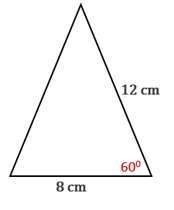Chapter 11.CT, Problem 15CTElementary Geometry For College St...

7th Edition
Alexander + 2 others
ISBN: 9781337614085

Solutions

Chapter
SectionElementary Geometry For College St...

7th Edition
Alexander + 2 others
ISBN: 9781337614085
Textbook Problem

Use one of the three forms for area (such as the form A = 1 2 b c sin ⁡ α ) to find the area of the triangle shown.Answer to the nearest whole number.

To determine

To find:

The area of the triangle using the formula A=12bcsinα to the nearest whole number.

Explanation

Given:

The triangle shown below.

Approach:

Formula to find the area of a triangle is A=12bcsinα.

Calculation:

From the given diagram,

Let b=8 cm, c=12 cm, and the angle included between b and c be α=600.

To find the area of the triangle, substitute b=8 cm, c=12 cm, and α=600 in the formula

A=

Still sussing out bartleby?

Check out a sample textbook solution.

See a sample solution

The Solution to Your Study Problems

Bartleby provides explanations to thousands of textbook problems written by our experts, many with advanced degrees!

Get Started

Find all possible real solutions of each equation in Exercises 3144. y3+64=0

Finite Mathematics and Applied Calculus (MindTap Course List)

Differentiate. F(t)=AtBt2+Ct3

Single Variable Calculus: Early Transcendentals, Volume I

Multiply the following numbers. 25.

Contemporary Mathematics for Business & Consumers

By determining the limit of Riemann sums, the exact value of dx is a) 7 b) 9 c) d) 18

Study Guide for Stewart's Single Variable Calculus: Early Transcendentals, 8th

The polar coordinates of the point P in the figure at the right are:

Study Guide for Stewart's Multivariable Calculus, 8th